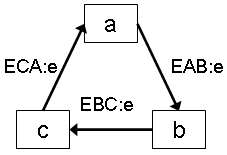# VIATRA2/Benchmarks/Sierpinsky triangles Benchmark

## Outdated page

The currently maintained wiki is available at http://wiki.eclipse.org/VIATRA

## Contents

### Benchmark description

This benchmark was presented as one of the case study of the 2007 Agtive tool contest. The goal of this case study is to measure the performance of graph transformation tools constructing Sierpinski triangles. The Sierpinski triangle is a fractal named after Waclaw Sierpinski who described it in 1915. Originally constructed as a mathematical curve, this is one of the basic examples of self-similar sets, i.e. it is a mathematically generated pattern that can be reproduced at any magnification or reduction.

An algorithm for obtaining arbitrarily close approximations to the Sierpinski triangle is as follows:

1. Start with an equilateral triangle with a base parallel to the horizontal axis.
2. Shrink the triangle by 1
3. make two copies, and position the three shrunk triangles so that each triangle touches each of the two other triangles at a corner.
4. Repeat step 2 with each of the smaller triangles.

### Metamodeling

From the programmers point of view, the most difficult part of implementing the Sierpinski triangle generator is to create the correct triangle ”finder mechanisms”. In our solution, we tried to adhere to the typing scheme found in the problem description by taking advantage of the multiple-inheritance support of the Viatra2 framework resulting the metamodel depicted in Fig. 1.
```entity ( node );
entity (a);   supertypeOf (node ,a);
entity (b);   supertypeOf (node ,b);
entity (c);   supertypeOf (node ,c);
entity (ac);  supertypeOf (a,ac );  supertypeOf (c,ac );
entity (ab);  supertypeOf (a,ab );  supertypeOf (b,ab );
entity (bc);  supertypeOf (b,bc );  supertypeOf (c,bc );
relation (e,node , node );```

As for the initial model it contained a simple triangle constructed of type A, B and a C nodes with their corresponding edges as shown in the following box:

```//Generating the 0 step model.
new(a(A));
new(b(B));
new(c(C));

new(node.e(E1,A,B));
new(node.e(E2,B,C));
new(node.e(E3,C,A));```

### Transformation details

This representation allowed us to create a very simple and elegant pattern illustrated in Fig. 2, where the left and right side describe the Viatra Textual Command Language (VTCL) representation and the graphical notation, respectively. Capital letters stand for variables, normal letters denote direct references to modelspace elements. For instance the expression (A) declares that the variable A is of type a. On the other hand, the expression node.e(ECA,C,A) means that the variable ECA refers to an edge of type node.e that points from the entity in C to A. The pattern in Fig. 2Fig.2 The pattern for matching a Sierpinsky triangle
matches a triangle with vertices type a,b,c, respectively. The order is granted by the direction of the arrows.
```pattern triangle(A,B,C,EAB,EBC,ECA) =
//Nodes
a(A); b(B); c(C);
//Edges
node.e(EAB,A,B); node.e(EBC,B,C);
node.e(ECA,C,A);```

As for the model manipulation we used simple built in new and delete operators as described in the following box:

```//delete the inner triangle
delete(EAB);
delete(EBC);
delete(ECA);

//The new nodes are created:
new(ab(AB) );
new(bc(BC) );
new(ac(AC) );

//The new edges are created:
new(node.e(EAAB,A,AB));
new(node.e(EABAC,AB,AC));
new(node.e(EACA,AC,A));

new(node.e(EABB,AB,B));
new(node.e(EBBC,B,BC));
new(node.e(EBCAB,BC,AB));

new(node.e(EACBC,AC,BC));
new(node.e(EBCC,BC,C));
new(node.e(ECAC,C,AC));```

TODO